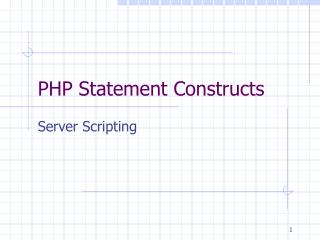DownloadDownload PresentationPHP Statement Constructs

# PHP Statement Constructs

Télécharger la présentation## PHP Statement Constructs

- - - - - - - - - - - - - - - - - - - - - - - - - - - E N D - - - - - - - - - - - - - - - - - - - - - - - - - - -
##### Presentation Transcript

1. PHP Statement Constructs Server Scripting

2. Basic Statement • All Statements end in a semicolon. • Statements are delimited from the HTML code by enclosing the code between • <?php ?> • Echo command is used to put text directly in the web page. • PHP code is mixed with the HTML code • Comments in PHP are delimited by • Either // for a comment on one line • /* and */ for blocks of comments.

3. Declaration of variables • Syntax var \$variablename; • Variables in PHP start with a dollar sign ("\$"). The value that a variable holds can be changed any time at all. • Var \$myname = “Fred”;

4. Constants • Syntax define (“Constant_Name”,”value”) • Define (“Const tax_rate”, 0.07) • The values maybe an integer, floating point or a string.

5. Declaring arrays • Syntax • \$variable = array (val1, val2, …, valn); • \$light = array (“red”, “yellow”, “green”); • To reference a specific element in the array • \$variable[pointervalue]—use parenthesis • The first position in an array is position 0.

6. Operators • Arithmetic operators are the same • Relational Operators • == -- one equal sign for equal comparison • != -- not equal • Other relational operators are the same • You may expand the relational statements by using the Logical Operators AND, OR • The not logical operator is represented by !

7. If Construct • Basic format • if (expresson) { true statements } else { false statements } end if

8. If Examples if (\$ans == “y”) or (\$ans == “Y”) { echo (“We will continue”); } else { echo (“We are stopping”); } if (\$total > 1.00) { \$total += (\$total * 0.07); }

9. More on If construct • The else clause and false statements are optional • The set of true or false statements are enclosed in braces • Each if construct must end with the brace delimiter • You may nest the if statements using the syntax if (condition) { true statement} elseif (condition) {true statement} else {false statements}

10. While Loop • Checks the condition and if the condition is true the loop executes. • The set of statements is enclosed in a set of brace • Format While (expression) { statements }

11. While example \$try_again = 5; while (\$try_again > 0) { echo (“Take another guess” ) ; \$try_again = \$try_again – 1; }

12. For Loop • Used when you know how many times you want the loop to execute. • This loop structure assumes the loop counter will be incremented by 1 at the end of the loop. • The statements for the loop are enclosed between the end of the count and the reserved word next

13. For Loop • Format for (start counter; stop condition; change counter) {statements }

14. FOR Example dim \$cntr; for (\$cntr = 10; \$cntr <= 20; \$cntr++) { echo (\$cntr); }